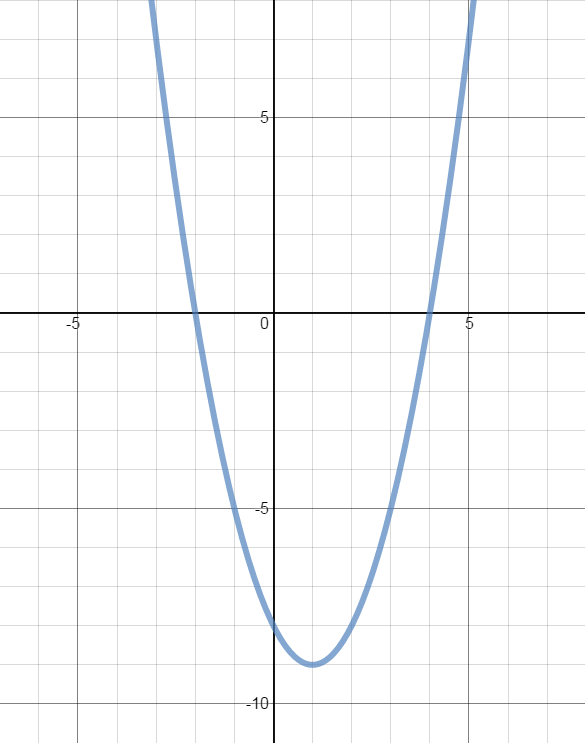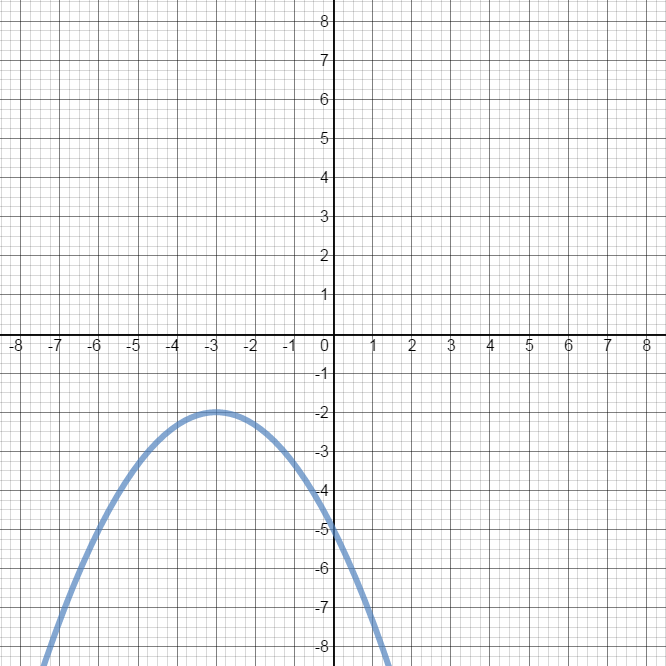# Characteristics of quadratic functions

### Characteristics of quadratic functions

Three properties that are universal to all quadratic functions: 1) The graph of a quadratic function is always a parabola that either opens upward or downward (end behavior); 2) The domain of a quadratic function is all real numbers; and 3) The vertex is the lowest point when the parabola opens upwards; while the vertex is the highest point when the parabola opens downward.

#### Lessons

• 1.
Determining the Characteristics of a Quadratic Function Using Various Methods

Determine the following characteristics of the quadratic function $y = -2x^2 + 4x + 6$:

• Opening of the graph

$y-$intercept

$x-$intercept(s)

• Vertex

• Axis of symmetry

• Domain

• Range

• Minimum/Maximum value

a)
Using factoring

b)
Using the quadratic formula

c)
Using completing the square

d)
Using the vertex formula

• 2.
From the graph of the parabola, determine the:
• vertex
• axis of symmetry
• y-intercept
• x-intercepts
• domain
• range
• minimum/maximum value
a)b)• 3.
Identifying Characteristics of Quadratic function in General Form: $y = ax^2 + bx+c$
$y = 2{x^2} - 12x + 10$ is a quadratic function in general form.

i) Determine:
• y-intercept
• x-intercepts
• vertex

ii) Sketch the graph.

• 4.
Identifying Characteristics of Quadratic Functions in Vertex Form: $y = a(x-p)^2 + q$
$y = 2{\left( {x - 3} \right)^2} - 8$ is a quadratic function in vertex form.

i) Determine:
• y-intercept
• x-intercepts
• vertex

ii) Sketch the graph.